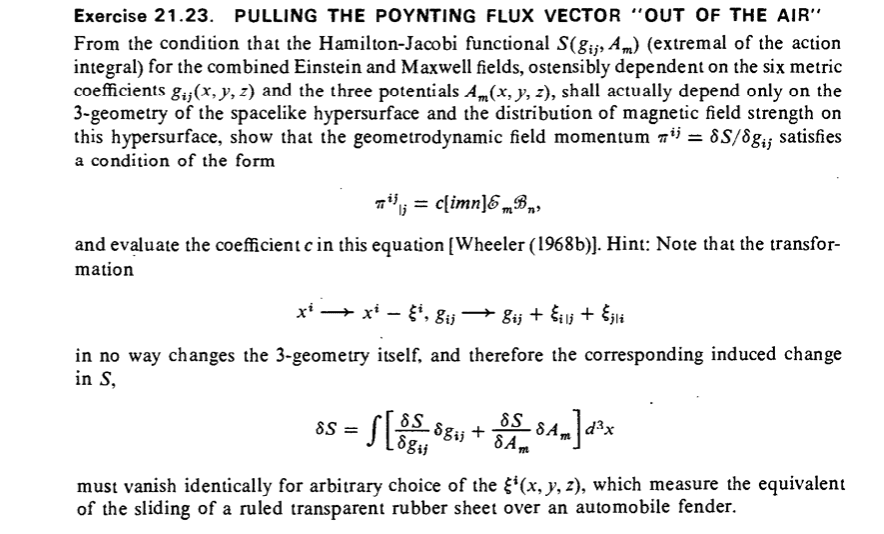# MTW Ex 21.23 Poynting Flux Vector 'out of the air'

• TerryW

#### TerryW

Gold Member
Homework Statement
I'm just looking for a bit of guidance with this, to check if I am on the right track
Relevant Equations
MTW (21.100) See Below
##4\pi\mathcal L = -\mathcal e \frac{\partial A_i }{\partial t} - \phi\mathcal E^i{}_{,i} -\frac{1}{2}N\gamma^{\frac{1}{2}}g_{ij}(\mathcal E^i \mathcal E^j +\mathcal B^i\mathcal B^j) +N^i [ijk]\mathcal E^i\mathcal B^j## MTW (21.100)

I'm trying to produce the result required by the problem below by using the Lagrangian Density above as the Hamilton-Jacobi functional ##(S(g_{ij},A_m)## referred to below. So far, I haven't been able to see a way forward which might end up with the required result.

At this stage, I'm just asking if MTW (21.100) is the appropriate functional and if it isn't, what should I be using?The answer to my question is yes. I found the key to the solution.